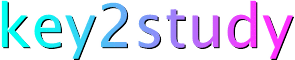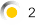Mathematics: BODMAS

The laws of precedence that apply to arithmetic also apply to algebraic expressions.  The order is:

• Brackets
• Of
• Division
• Multiplication
• Subtraction

or BODMAS!

As an example, let's suppose that we need to find the value of:

5 × 2  9

Multiplication is performed before addition and subtraction thus:

5 × 2  9 = 10  9 = 1

Now try using the BODMAS rule to work out the value of each of the expressions below. Then enter the expression into the text box and click on Calculate to check your answer.

You can enter numbers, maths operators and brackets using +, -, /, ( and ). Note that the computer requires you to use * instead of x when you want to multiply two numbers. Thus, 3 x 4 is entered as 3 * 4.

 (a) 5 x 4 + 3 Enter this as:  5 * 4 + 3, and so on (b) 5 x (4 + 3) (c) 5 x 2 + 3 + 5 x 4 (d) (5 x 2) + (3 + 5) x 4 (e) ((5 x 2) + (3 + 5)) x 4 (f) 5 x (2 + 3) + (5 x 4) (g) 5 x ((2 + 3) + (5 x 4))